# Area of Parallelograms and Triangles Worksheet### MCQ on Areas of parallelograms and Triangles

Ques. A parallelogram ABCD and a rectangle ABPQ are on the same base AB and between the same parallels AB and CQ. If AB = 8 cm and AQ = 6 cm, find the area of ||gm ABCD.
(a) 52 cm2
(b) 46 cm2
(c) 48 cm2
(d) 58 cm2

Ans. (c)

Ques. In the given figure, PQS = SRQ, QPR = SRQ,  U is a point on QR such that QPU = 300, then QUP is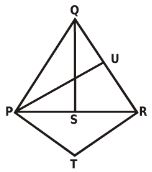(a) 40o
(b) 50o
(c) 60o
(d) 70o

Ans. (c)

Ques. One side of a rectangular field is 15 m and one of its diagonals is 17 m. Find the area of the field.
(a)

120 m2
(b) 80 m2
(c) 90 m2
(d) 70 m2
Ans. (a)

Ques. In a ABC, the medians AD, BE and CF intersects at G. If AD = 7.2 cm, then AG is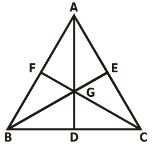(a) 4.8 cm
(b) 2.4 cm
(c) 3.6 cm
(d) 1.7 cm

Ans. (a)

Ques. The area of the parallelogram formed by the lines y = mx, y = mx + 1, y = nx and y = nx + 1 equals
(a) |m + n| / (m – n)2
(b) 2 / | m + n |
(c) 1 / | m + n|
(d) 1 / |m – n|

Ans. (d)

Ques. In a parallelogram ABCD ∠D = 60o then the measurement of ∠A
(a) 120o
(b) 65o
(c) 90o
(d) 75o

Ans. (a)

Ques. In the figure below, PQ!!SR, If x =  and y = , then the values of x, y, z are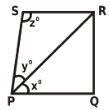(a) 36, 60, 84
(b) 45, 25, 110
(c) 46, 50, 84
(d) None of these

Ans. (a)

Ques. Find the area of a square, one of whose diagonals is 3.8 m long.
(a) 8.25 m2
(b) 8.22 m2
(c) 7.25 m2
(d) 7.22 m2

Ans. (d)

Related: Number series questions

Ques. In ABC, AD is a median and P is a point is AD such that AP : PD = 1 : 2 then the area of ABP =
(a) 1/3 x Area of ABC
(b) 2/3 x Area of ABC
(c) 1/3 x Area of ABC
(d) 1/6 x Area of ABC

Ans. (d)

Ques. Medians BD and CE of triangle ABC are perpendicular, BD = 8 and CE = 12. The area of triangle ABC is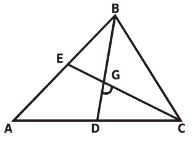(a) 24
(b) 32
(c) 48
(d) 64

Ans. (d)

Ques. Find the area a rhombus, the lengths of whose diagonals are 16 cm and 24 cm respectively.
(a) 92 cm2
(b) 192 cm2
(c) 108 cm2
(d) 168 cm2

Ans. (b)

Ques. Find the cost of carpeting a room 13 m long and 9 m broad with a carpet 75 cm wide at the rate of \$12.40 per square metre.
(a) 1944.40
(b) 1934.40
(c) 1930.40
(d) 1940.40

Ans. (b)

Ques. If x2 + y2 + z2 = xy + yz + zx, then the triangle is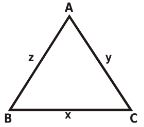(a) Isosceles
(b) right angled
(c) equilateral
(d) scalene

Ans. (c)

Ques. The resultant of three forces represented in magnitude and direction by the sides of a triangle ABC taken in order with BC = 5 cm, CA = 5 cm, and AB = 8 cm, is a couple of moment
(a) 12 unit
(b) 24 unit
(c) 36 unit
(d) 16 unit

Ans. (b)

Ques. The sum of the interior angles of polygon is three times the sum of its exterior angles. Then numbers of sides in polygon is
(a) 6
(b) 7
(c) 8
(d) 9

Ans. (c)

Ques. In the given figure, ABC is a triangle and D is a point on AC, given that ABC = 65o, BDC = 2ABD and BDA = 3DBC. Then BCD is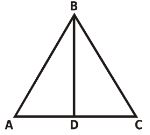(a) 100o
(b) 105o
(c) 110o
(d) 120o

Ans. (a)

Ques. A room is half as long again as it is broad. The cost of carpeting the at \$5 per sq. m is \$270 and the cost of papering the four walls at \$10 per m2 is \$1720. If a door and 2 windows occupy 8 sq. m, find the dimensions of the room.
(a) 19 m
(b) 13 m
(c) 7 m
(d) 6 m

Ans. (d)

Ques. In the given triangle DEF, points A,B and C are taken on DE, DF and EF respectively such that EC = AC and CF = BC. If angle D = 40 degrees, then  the angle of ACB in degrees is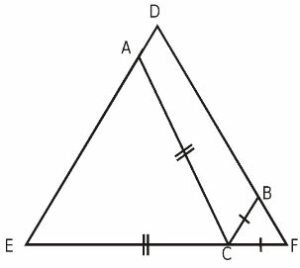(a) 140
(b) 70
(c) 100
(d) None of these

Ans. (c)

Ques. ABCD is parallelogram. If AB = 3.6 cm and altitude corresponding to sides AB and AD are respectively 5 cm and 4 cm, then AD will be
(a) 5.5 cm
(b) 3.5 cm
(c) 2.5 cm
(d) 4.5 cm

Ans. (d)

Que. ABCD is a parallelogram, if the two diagonals are equal, then the measure of ABC is
(a) 90o
(b) 45o
(c) 60o
(d) 120o

Ans. (a)

Ques. In the figure (not drawn to scale) given below,if AD = CD = BC, and ,  then ∠DBC is
(a) 32o
(b) 84o
(c) 64o
(d) Cannot be determined

Ans. (c)

Ques. If the equations of opposite sides of a parallelogram are x2 – 7x + 6 = 0 and y2 – 14y + 40 = 0, then the equation of its one diagonal is
(a) 6x + 5y + 14 = 0
(b) 6x – 5y + 14 = 0
(c) 5x + 6y + 14 = 0
(d) 5x – 6y + 14 = 0

Ans. (b)

Ques. In the following triangle ABC, the angles  x, y, z are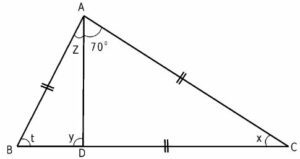(a) 40o, 110o, 30o
(b) 100o, 30o, 50o
(c) 50o, 70o, 60o
(d) 80o, 60o, 30o

Ans. (a)

Ques. The sides of a triangle are in the ratio 3 : 5 : 7 and its perimeter is 30 cm. The length of the greatest side of the triangle in cm is
(a) 6
(b) 10
(c) 14
(d) 16

Ans. (c)

Ques. If each side of a square is increased by 25%, find the percentage change in its area.
(a) 47.25%
(b) 66.25%
(c) 62.25%
(d) 56.25%

Ans. (d)

Ques. In the figure below, CF and DG are the bisectors of ∠BCD and ∠BDC  respectively. then ∠GFC is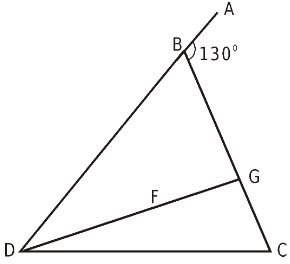(a) 50o
(b) 60o
(c) 65o
(d) 75o

Ans. (c)

Related: complex number test

Ques. In ABC if D is a point in BC and divides it the ratio 3 : 5 i.e., if BD : DC = 3 : 5 then, ar (ADC) : ar(ABC) = ?
(a) 3 : 5
(b) 3 : 8
(c) 5 : 8
(d) 8 : 3

Ans. (c)

Ques. The perimeters of two squares are 40 cm and 32 cm. Find the perimeter of a third square whose area is equal to the difference of the areas of the two squares.
(a) 32 cm
(b) 28 cm
(c) 24 cm
(d) 20 cm

Ans. (c)

Ques. In the given figure, B = C = 65° and D = 30°, then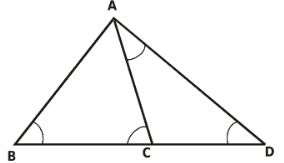(a) BC < CA < CD
(b) BC < CA, CA > CD
(c) BC > CA > CD
(d) BC > CA, CA < CD

Ans. (a)

Ques. How many triangles can be drawn by means of 9 non-collinear points
(a) 84
(b) 72
(c) 144
(d) 126

Ans. (a)

Ques. The angle between the bisectors of the two acute angles of a right angle triangle is
(a) 120o
(b) 135o
(c) 112½o
(d) 90o

Ans. (b)

Ques. Find the area of a right-angled triangle whose base is 12 cm and hypotenuse is 13cm.
(a) 30 cm2
(b) 25 cm2
(c) 35 cm2
(d) 20 cm2

Ans. (a)

Ques. In the figure the measure of A is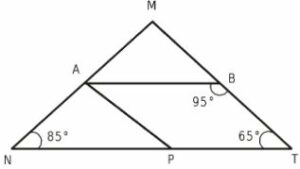(a) 95°
(b) 105°
(c) 65°
(d) 35°

Ans. (c)

Ques. Find the area of trapezium whose parallel sides are 8 cm and  6 cm respectively and the distance between these sides is 8 cm.
(a) 56 cm2
(b) 52 cm2
(c) 58 cm2
(d) 54 cm2

Ans. (a)

Related: hardware computer quiz questions

Ques. The area of trapezium whose parallel sides are 9cm and 6cm respectively and distance between these sides is 8 cm will be
(a) 50 cm2
(b) 60 cm2
(c) 70 cm2
(d) 80 cm2

Ans. (b)

Ques. If EFC = y°,  FEB = x°,  ACD = 128°, AB = AE, ABEF then angles x° and y° are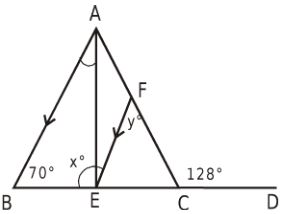(a) x = 58° ; y = 110°
(b) x = 110° ; y = 58°
(c) x = 110° ; y = 180°
(d) x = 68° ; y = 110°

Ans. (b)

Ques. Area of rhombus whose diagonals are 16cm and 24cm will be
(a) 182 cm2
(b) 202 cm2
(c) 92 cm2
(d) 192 cm2

Ans. (d)

Ques. A room 5m 55cm long and 3m 74 cm broad is to be paved with square tiles. Find the least number of square tiles required to cover the floor.
(a) 173
(b) 174
(c) 175
(d) 176

Ans. (d)

Ques. If B < A and C <D, then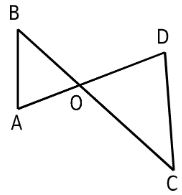Ans. (b)

Ques. If the diagonal of a rectangle is 17 cm long and its perimeter is 46 cm, find the area of the rectangle.
(a) 100 cm2
(b) 120 cm2
(c) 80 cm2
(d) 150 cm2

Ans. (c)

Question: The number of triangles that can be formed by 5 points in a line and 3 points on a parallel line, is
(a) 8C3
(b) 8C35C3
(c) 8C35C3 – 1
(d) none of these

Ans. (c)

Ques. If AB = 13x – 50 ; AE = 20 – 11y ; DF = 50 – 11x ;  CF = 13y – 24, AB = AE, FC = DF, then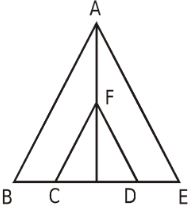(a) x > y
(b) x = y
(c) x < y
(d) x > y

Ans. (c)

Related: Cube roots worksheet

Ques. Find the length of a rope by which a cow must be tethered in order that it may be able to graze an area of 9856 sq. metres.
(a) 58 m
(b) 48 m
(c) 56 m
(d) 52 m

Ans. (c)

Ques. In a parallelogram ABCD, AB = 8 cm. The altitudes corresponding to sides AB and AD are respectively 4 m and 5 cm. Find AD.
(a) 6.4 cm
(b) 6.6 cm
(c) 6.5 cm
(d) 6.2 cm

Ans. (a)

Ques. If the length of a certain rectangle is decreased by 4 cms and the width is increased by 3 cms, a square with the same area as the original rectangle would result. Find the perimeter of the original rectangle.
(a) 50 cms
(b) 51 cms
(c) 52 cms
(d) 53 cms

Ans. (a)

Ques. The difference between two parallel sides of a trapezium is 4 cms. perpendicular distance between them is 19 cms. If the area of the trapezium is 475 find the lengths of the parallel sides.
(a) 26 cms, 25 cms
(b) 27 cms, 23 cms
(c) 28 cms, 25 cms
(d) 25 cms, 22 cms

Ans. (b)

Ques. In two triangles, the ratio of the areas is 4 : 3 and the ratio of their heights is 3 : 4. Find the ratio of their bases.
(a) 6 : 7
(b) 16 : 9
(c) 4 : 5
(d) 16 : 15

Ans. (b)

Related: Train questions

Ques. The length of a rectangle is twice its breadth. If its length is decreased by 5 cm and breadth is increased by 5 cm, the area of the rectangle is increased by 75 sq. cm. Find the length of the rectangle.
(a) 15.5 cm
(b) 17 cm
(c) 22 cm
(d) 20 cm

Ans. (d)

Ques. The diagonals of two squares are in the ratio of 2 : 5. Find the ratio of their areas.
(a) 3 : 4
(b) 4 : 5
(c) 4 : 25
(d) 6 : 11

Ans. (c)

Ques. Find the length of the altitude of an equilateral triangle of side 33 cm.
(a) 5.5 cms
(b) 6.5 cms
(c) 4.5 cms
(d) 7.5 cms

Ans. (c)

Ques. In measuring the sides of a rectangle, one side is taken 5% in excess, and the other 4% in deficit. Find the error percent in the area calculated from these measurements.
(a) 0.8%
(b) 1.8%
(c) 1.2%
(d) 1.0%

Ans. (a)

Ques. Find the area of a triangle whose sides measure 13 cm, 14 cm and 15 cm.
(a) 78 cm2
(b) 86 cm2
(c) 84 cm2
(d) 82 cm2

Ans. (c)

Related: currency of countries

Ques. The base of  a parallelogram is twice its height.  If the area of the parallelogram is 72 sq. cm, find its height.
(a) 8 cms
(b) 6 cms
(c) 9 cms
(d) 5 cms

Ans. (b)

Ques. A rectangular grassy plot 110 m. by 65 m has a gravel path 2.5 m wide all round it on the inside. Find the cost of gravelling the path at 80 cents per sq. metre.
(a) \$670
(b) \$680
(c) \$640
(d) \$650

Ans. (b)